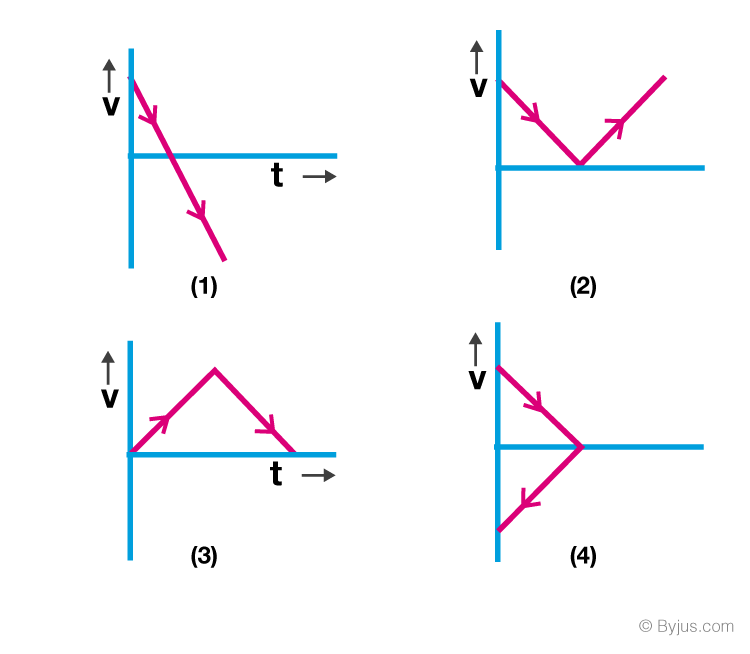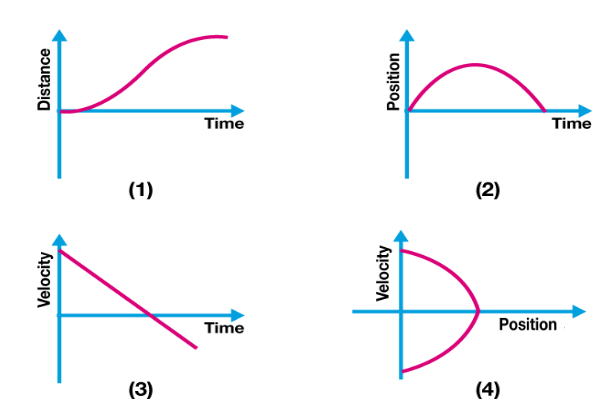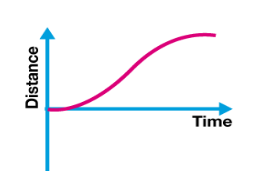# JEE Main Kinematics 1D Previous Year Questions with Solutions

We find objects in motion all around us. Everything from a moving cricket ball to the planets and galaxies is in motion. Kinematics is the branch of physics that describes the position and motion of objects as a function of time. Kinematic analysis is used for measuring the quantities related to motion.The important quantities in Kinematics are displacement, velocity, acceleration and time. A lot of questions on the motion of the object are answered in KInematics like when a ball is thrown at a certain angle where it will land and many more. The quantities like acceleration help in the study of other concepts like force.

Kinematics is used in the study of the motion of celestial bodies, mechanical engineering, robotics, etc.

All the important questions with detailed explanations of the answers from the topic Kinematics ID, asked in previous years’ question papers of JEE Main are included in this page. IIT aspirants are advised to go through this page to have an idea about the possible questions asked from this topic.

## JEE Main Past Year Questions With Solutions on Kinematics 1D

Q1: A piece of wood of mass 0.03 kg is dropped from the top of a 100 m height building. At the same time, a bullet of mass 0.02 Kg is fired vertically upward, with a velocity 100 m/s, from the ground. The bullet gets embedded in the wood. Then the maximum height to which the combined system reaches above the top of the building before falling below is (g = 10 m/s)

1. 10 m
2. 30 m
3. 20 m
4. 40 m

Solution

Suppose both collide at the point P after time t. Time taken for the particles to collide,

t = d/vrel = 100/100 = 1s

Speed of wood just before collision =gt = 10m/s

Speed of bullet just before collision

v -gt = 100 -10 = 90 m/s

Before

0.03 kg ↓ 10 m/s

0.02 kg ↑ 90 m/s

After

↑ v

0.05 kg

Now, the conservation of linear momentum just before and after the collision

-(0.03)(10) + (0.02)(90) = (0.05)v ⇒ v = 30 m/s

The maximum height reached by the body a = v2/2g

= (30)2/2(10)

= 45 m

(100 -h) = ½ gt2 = ½ x 10 x1 ⇒h = 95 m

Height above tower = 40 m

Q2: A passenger train of length 60 m travels at a speed of 80 km/hr. Another freight train of length 120 m travels at a speed of 30 km/hr. The ratio of times taken by the passenger train to completely cross the freight train when: (i) they are moving in the same direction, and (ii) in the opposite direction, is

(a) 25/11

(b) 3/2

(c) 5/2

(d) 11/5

Solution:

The total distance to be travelled by train is 60 + 120 = 180 m.

When the trains are moving in the same direction, the relative velocity is v1 – v2 = 80 – 30 = 50 km hr–1.

So time taken to cross each other, t1 = 180/(50 x 103/3600) = [(18 x 18)/25] s

When the trains are moving in opposite direction, relative velocity is |v1 – (–v2 )| = 80 + 30 = 110 km hr–1

So time taken to cross each other

t2= 180/(110 x 103/3600) = [(18 x 36)/110] s

t1/t2= [(18 x 18)/25] / [(18 x 36)/110] = 11/5

Q3: A particle has an initial velocity $3{\hat{i}}+4{\hat{j}}$ and an acceleration of $0.4{\hat{i}}+0.3{\hat{j}}$. Its speed after 10s is

1. 7 units
2. 8.5 units
3. 10 units
4. $7\sqrt{2}$ units

Solution:

v = u + at

u = 3i + 4j + (0.4 i + 0.3 j) x 10

= 3i + 4j + 4i + 3i

u = 7i + 7j

$\left | \vec{u} \right |= \sqrt{7^{2}+7^{2}}$

Answer: (d) $7\sqrt{2}$ units

Q4: An automobile, travelling at 40 km/h, can be stopped at a distance of 40 m by applying brakes. If the same automobile is travelling at 80 km/h, the minimum stopping distance, in metres, is (assume no skidding)

(a) 100 m

(b) 75 m

(c) 160 m

(d) 150 m

Solution :

Using v2 = u2 – 2as

0 = u2 – 2as

S = u2 /2a

S1/S2 = u12/u22

S2 = (u12/u22)S1 = (2)2(40) = 160 m

Q5: A body is thrown vertically upwards. Which one of the following graphs correctly represents the velocity vs time?Solution:

Velocity at any time t is given by

v = u + at

v = v(0) + (-gt)

v = -gt

Straight line with negative slope

Q6: All the graphs below are intended to represent the same motion. One of them does it incorrectly. Pick it up.Solution: In this question option (2) and (4) are the corresponding position-time graph and velocity – position graph of the option (3) and its distance-time graph is given asHence, the answer is given as (1)

Q7. A parachutist after bailing out falls 50 m without friction. When the parachute opens, it decelerates at 2 m/s2. He reaches the ground with a speed of 3 m/s. At what height, did he bailout?

(a) 293 m

(b) 111 m

(c) 91 m

(d) 182 m

Solution:

Initially, the parachutist falls under gravity u 2 = 2ah = 2 × 9.8 × 50 = 980 m2s –2

He reaches the ground with speed = 3 m/s,

a = –2 m s–2 ⇒ (3)2 = u 2 – 2 × 2 × h1

9 = 980 – 4 h1

h1 = 971/4

h1 = 242.75 m

Total height = 50 + 242.75 = 292.75 = 293 m.

Q8: A car, starting from rest, accelerates at the rate f through a distance s, then continues at a constant speed for time t and then decelerates at the rate f/2 to come to rest. If the total distance traversed in 15 s, then

(a) s= ½ft2

(b) s= (¼)ft2

(c) s = ft

(d) s= (1/72)ft2

Solution:

For the first part of the journey, s = s1,

s1 = ½ ft12………………………(1)

v = f t1 …………………………(2)

For second part of journey,

s2 = vt or s2 = f t1 t ……………(3)

For the third part of the journey,

s3 = ½(f/2)(2t1)2= ½ x ft12

s3 = 2s1 = 2s ………………….(4)

s1 + s2 + s3 =15s

Or s + ft1t + 2s = 15s

ft1t = 12 s

From (1) and (5) we get

(s/12 s )= ft12/(2 x ft1t)

Or t1 = t/6

Or s= ½ ft12

s= ½ f(t/6)2

s= ft2/72

Q9: An automobile travelling with a speed of 60 km/h, can brake to stop within a distance of 20 m. If the car is going twice as fast, i.e., 120 km/h, the stopping distance will be

(a) 20 m

(b) 40 m

(c) 60 m

(d) 80 m

Solution:

Let a be the retardation for both the vehicles.

For automobile, v 2 = u 2 – 2as

u1 2 – 2as1 = 0

u1 2 = 2as1

Similarly for car, u2 2 = 2as2

(u2/u1)2 = s2/s1 = (120/60)2 = s2/20

S2 = 80 m

Q10: A ball is released from the top of a tower of height h metre. It takes T second to reach the ground. What is the position of the ball in T/3 second?

(a) (h/9)metre from the ground

(b) (7h/9) metre from the ground

(c) (8h/9) metre from the ground

(d) (17h/18) metre from the ground

Solution:

Equation of motion

s= ut + gt2

h = 0 + ½ gT2

Or 2h = gT2………(1)

After T/3 sec, s = 0 +½ x g(T/3)2= gT2/18

18 s = gT2 …………(2)

From (1) and (2), 18 s = 2h

S = (h/9) m from top.

Height from ground = h – h/9 = (8h/9) m

Answer: (c) (8h/9) metre from the ground

Q10: Which of the following statements is false for a particle moving in a circle with a constant angular speed?

(a) The velocity vector is tangent to the circle

(b) The acceleration vector is tangent to the circle

(c) The acceleration vector points to the centre of the circle

(d) The velocity and acceleration vectors are perpendicular to each other

Answer: (b) The acceleration vector acts along the radius of the circle. The given statement is false.

Q11: From a building, two balls A and B are thrown such that A is thrown upwards and B downwards (both vertically). If vA and vB are their respective velocities on reaching the ground, then

(a) vB > vA

(b) vA = vB

(c) vA > vB

(d) their velocities depend on their masses

Solution

Ball A projected upwards with velocity u, falls back with velocity u downwards. It completes its journey to the ground under gravity.

vA 2 = u2 + 2gh …(1)

Ball B starts with downwards velocity u and reaches ground after travelling a vertical distance h vB 2 = u2 + 2gh …(2)

From (1) and (2)

vA = vB

Q12: If a body loses half of its velocity on penetrating 3 cm in a wooden block, then how much will it penetrate more before coming to rest?

(a) 1 cm

(b) 2 cm

(c) 3 cm

(d) 4 cm

Solution

For first part of penetration, by equation of motion,

(u/2)2 = u2 -2a(3)

3u2 = 24a ⇒ u2 = 8a …(1)

For latter part of penetration,

0= (u/2)2 -2ax

or u2 = 8ax……………(2)

From (1) and (2)

8ax = 8a x = 1 cm

Q13: A car is standing 200 m behind a bus, which is also at rest. The two start moving at the same instant but with different forward accelerations. The bus has acceleration 2m/s2 and the car has acceleration 4m/s2. The car will catch up with the bus after a time of

(a) 120 s

(b) 15 s

(c) $2\sqrt{2}$ s

(d) 110 s

Solution

Acceleration of car, aC = 4 m s–2

Acceleration of bus, aB = 2 m s–2

Initial separation between the bus and car, sCB = 200 m

Acceleration of car with respect to bus, aCB = aC – aB = 2 m s–2

Initial velocity uCB = 0, t =?

As, sCB = uCB × t + 1 /2 aCB t2

200 = 0 × t + ½× 2 × t2

i.e., t2 = 200

t = $2\sqrt{2}$

Answer: (c) $2\sqrt{2}$ s

Q14: From a tower of height H, a particle is thrown vertically upwards with a speed u. The time taken by the particle, to hit the ground is n times that taken by it to reach the highest point of its path. The relation between H, u and n is

(a) gH = (n – 2)u2

(b) 2gH = n 2u2

(c) gH = (n – 2)2u2

(d) 2gH = nu2(n – 2)

Solution

Time taken by the particle to reach the topmost point is,

t =u/g … (1)

Time taken by the particle to reach the ground = nt

Using, s = ut + ½ at2

-H = u(nt) – ½ g(nt)2

-H = u x n (u/g) = ½ g2(u/g)2 [using (1)]

⇒-2gH = 2nu2 – n2u2

⇒ 2gH = nu2(n -2)

Answer: (d) 2gH = nu2(n – 2)

Q15: For a tower of height H, a particle is thrown vertically upwards with a speed u. The time taken by the particle to hit the ground is n times that taken by it to reach the highest point of its path. The relation between H,u and n is

1. 2gH = nu2 (n-2)
2. gH = (n-2)u2
3. 2gH = n2u2
4. gH = (n – 2)2u2

Solution:

Time to reach highest point = t = u/g

Time to reach ground = nt

S = ut + ½ at2

-H = u(nt) – ½ g (nt)2

2gH = nu2 (n-2)

Answer: (a) 2gH = nu2 (n-2)